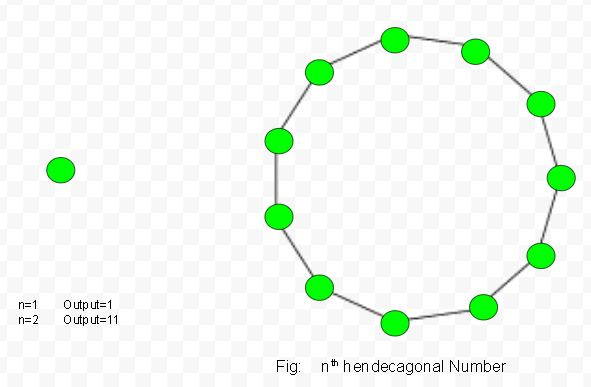# Hendecagonal number

Given a number n, the task is to find the nth Hendecagonal number.
A Hendecagonal number is a figurate number that extends the concept of triangular and square numbers to the decagon (Eleven -sided polygon). The nth hendecagonal number counts the number of dots in a pattern of n nested decagons, all sharing a common corner, where the ith hendecagon in the pattern has sides made of i dots spaced one unit apart from each other.
Examples:

Input : 2
Output :11
Input :6
Output :141Formula for nth hendecagonal number :## C++

 // C++ program to find nth // Hendecagonal number #include  using namespace std;   // Function to find // Hendecagonal number int hendecagonal_num(int n) {     // Formula to calculate nth     // Hendecagonal number     return (9 * n * n - 7 * n) / 2; }   // Driver Code int main() {     int n = 3;     cout << n << "rd Hendecagonal number: ";     cout << hendecagonal_num(n);     cout << endl;     n = 10;     cout << n << "th Hendecagonal number: ";     cout << hendecagonal_num(n);       return 0; }

## C

 // C program to find nth // Hendecagonal number #include    // Function to find // Hendecagonal number int hendecagonal_num(int n) {     // Formula to calculate nth     // Hendecagonal number     return (9 * n * n - 7 * n) / 2; }   // Driver Code int main() {     int n = 3;     printf("%drd Hendecagonal number: ",n);     printf("%d\n",hendecagonal_num(n));       n = 10;     printf("%dth Hendecagonal number: ",n);     printf("%d\n",hendecagonal_num(n));       return 0; }   // This code is contributed by kothavvsaakash.

## Java

 // Java program to find nth // Hendecagonal number import java.io.*;   class GFG {       // Function to find // Hendecagonal number static int hendecagonal_num(int n) {     // Formula to calculate nth     // Hendecagonal number     return (9 * n * n -              7 * n) / 2; }   // Driver Code public static void main (String[] args) { int n = 3; System.out.print(n + "rd Hendecagonal " +                              "number: "); System.out.println(hendecagonal_num(n));   n = 10; System.out.print(n + "th Hendecagonal " +                               "number: "); System.out.println(hendecagonal_num(n)); } }   // This code is contributed by ajit

## Python3

 # Program to find nth # Hendecagonal number   # Function of Hendecagonal  # number  def hendecagonal_num(n) :           # Formula to calculate nth     # Hendecagonal number &     # return it into main function.           return (9 * n * n -             7 * n) // 2   # Driver Code if __name__ == '__main__' :               n = 3     print(n,"rd Hendecagonal number : " ,                      hendecagonal_num(n))       n = 10     print(n,"th Hendecagonal number : " ,                      hendecagonal_num(n))   # This code is contributed by ajit

## C#

 // C# program to find nth // Hendecagonal number using System;   class GFG { // Function to find // Hendecagonal number static int hendecagonal_num(int n) {     // Formula to calculate nth     // Hendecagonal number     return (9 * n * n - 7 * n) / 2; }   // Driver Code static public void Main () {     int n = 3;     Console.Write(n +                   "rd Hendecagonal number: ");     Console.WriteLine( hendecagonal_num(n));       n = 10;     Console.Write(n +                   "th Hendecagonal number: ");     Console.WriteLine( hendecagonal_num(n));     } }   // This code is contributed by aj_36

## PHP

 

## Javascript

 

Output :

3th Hendecagonal number: 30
10th Hendecagonal number: 415

Time Complexity: O(1)
Auxiliary Space: O(1)
Reference: https://en.wikipedia.org/wiki/Polygonal_number

Feeling lost in the world of random DSA topics, wasting time without progress? It's time for a change! Join our DSA course, where we'll guide you on an exciting journey to master DSA efficiently and on schedule.
Ready to dive in? Explore our Free Demo Content and join our DSA course, trusted by over 100,000 geeks!

Previous
Next